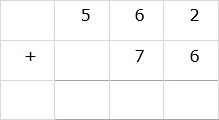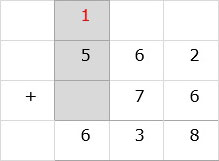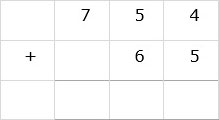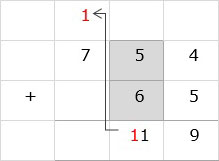# Addition With Carry to the Hundreds Place

Find the sum 562 + 76

### Solution

Step 1:

We line up the numbers vertically.Step 2:

We add the digits in ones column. 2 + 6 = 8. 8 is written below ones column.Step 3:

Then we add the digits in tens column. 6 + 7 = 13. 3 is written below tens column. 1 is carried over to hundreds column.Step 4:

We add 5 and 1 in hundreds column. 5 + 1 = 6. 6 is written below hundreds column to give the final sum as 638.Find the sum 754 + 65

### Solution

Step 1:

We line up the numbers vertically.Step 2:

We add the digits in ones column. 4 + 5 = 9. 9 is written below ones column.Step 3:

Then we add the digits in tens column. 5 + 6 = 11. 1 is written below tens column. 1 is carried over to hundreds column.Step 4:

We add 7 and 1 in hundreds column. 7 + 1 = 8. 8 is written below hundreds column to give the final sum as 819.